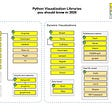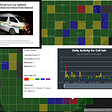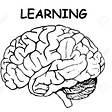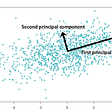# Regression and Causal Inference: How Causal Graphs Helped Me Overcome 3 Key Misconceptions

Regression can be used for two purposes: predictive modeling and causal inference. However, based on my experience and understanding, introductory/intermediate statistics/data science courses focus almost entirely on the application of regression for predictive purposes. This makes some sense in terms of preparing students for a successful career in the data science industry. After all, under relatively stable real-world conditions, as long as you can predict the value of Y based on the value of X with a reasonable amount of precision, you may not have much incentive to investigate whether X causes Y, or Y causes X, or both X and Y are caused by a common factor Z.

Things get complicated when we try to interpret the coefficients of a correlational statistical model — estimated by disregarding the underlying causal relationships among the relevant variables — in causal terms. Such practices can lead to a continued misunderstanding of a phenomenon and ineffective/less effective policy suggestions.

Perhaps, the introductory/intermediate statistics/data science courses can do more to provide a fundamental (yet deeper) understanding of causal inference along with the knowledge of statistical inference. In fact, some of the complicated concepts can be easily explained by using causal graphs, known as Directed Acyclic Graphs or DAGs, as shown in the book “Causal Inference in Statistics: A Primer” by Pearl, Glymour, and Jewell.

In this article, I will try to explain how a basic understanding of causal graphs helped me overcome three misconceptions about causal inference from estimating empirical models using linear regression.

Example

Let us assume, a researcher is trying to investigate the causal effect of a treatment X on an outcome Y. She estimates the following model using some non-experimental data:

Y = b0 + b1 * X + e (where, e is the error term)

Misconception #1: You need to control for all other causes of the Outcome (Y) other than the Treatment (X)

Somehow, many people come up with the understanding that to estimate the causal effect of a treatment X on an outcome Y, you need to control for all other causes of Y (by adding all these relevant variables to the model); and so, if you have not added all these variables, you cannot suggest that the coefficient of X (b1) is an estimator of the causal effect of X on Y.

In reality, for causal inference, in our model, we need to add only those variables which are the common causes of both the treatment and the outcome. These variables are called “confounding variables” or simply “confounders”.

In the case of our hypothetical researcher, let us pretend that there are only two common causes (A and B) of the treatment and the outcome, and the causal relationships among the variables of interest can be shown as in the following causal graph:

In graph I, e represents all other causes of the outcome Y except A, B, and X. Controlling for A and B (i.e. adding A and B to the model as predictors along with X), closes the two non-causal paths (shown in red; also known as backdoor paths) from X to Y: X ← A →Y and X ← B →Y, and only the causal path XY remains open for information to flow from X to Y.

As long as our researcher has data on A, B, X, and Y, she can estimate the causal effect of X on Y (even if she does not have any data on e) from the following model:

Y = b0 + b1 * X + b2 * A + b3* B + e (where, e is the error term)

Given the causal graph is correct, in the above model, b1 is a consistent estimator of the causal effect of X on Y.

Of course, we need to make some more theoretical assumptions — for example, stable unit treatment value assumption (SUTVA), positivity, treatment effect homogeneity, etc. — for valid causal inference from a model estimated using observational data; but we will keep things simple here and focus only on how causal graphs can guide our selection of variables to be included in the model.

Misconception #2: Throw the kitchen sink at your model!

Sometimes, people suggest you add more, and more, and more…….., and more “control variables” to your model. Apparently, the assumption is that all these control variables are confounders and if you do not add all of them, you cannot infer any causal relationship.

But, are all of these control variables really confounders? If they are, yes, you should add them to your model. However, you need to be cognizant of two other types of variables — mediators and colliders — controlling for which can make your causal estimate inconsistent.

In graph II, there are 3 causal paths from X to Y: two indirect paths (X → A → Y and X → B → Y) and a direct path (XY). If this causal graph is correct, then A and B are “mediators” (not confounders), and controlling for either A or B will almost certainly lead to a biased and inconsistent estimation of the total causal effect of X on Y.

Another possibility, as shown in graph III, is that both A and B are common effects of X and Y. In this case, A and B are called “colliders”, and interestingly, if we control for nothing, the non-causal paths (shown in yellow) X → A ← Y and X → B ← Y are automatically closed (that is no information flows through these paths). However, if we control for A and B, then the paths are opened, and we are highly likely to get a biased and inconsistent estimate of the causal effect of X on Y.

As a simple rule of thumb, we may remember: if we are interested in estimating the total causal effect of a treatment, we should control for variables that exist before treatment assignment (likely confounders) and should not control for variables that exist after treatment assignment (likely mediators and colliders). So, knowledge of temporal precedence can be useful for deciding on which variables to control for. However, there are situations in which the above-mentioned rule will not work(for example, in the case of M-bias). The problem is that whether a variable is a confounder or a mediator or a collider depends on the path in which it is located; that is, the same variable can be a confounder in one path and a collider/a mediator in another path! So, the best idea is to figure out all the causal and non-causal paths from X (treatment) to Y (outcome) and then depending on the situation, decide on which variables to control for in the model. I discuss the issue succinctly in another blog:

Misconception #3: You need to control for all the confounders

Earlier, we mentioned that common causes of both the treatment and the outcome are called confounders. Now, let us look at the following causal graph:

Here, the two non-causal paths between X and Y are: X← A →Y and X← A ← B →Y. We may claim that both A and B are confounders as they are common causes of X and Y. Interestingly, in this case, controlling for only A is sufficient to close both non-causal paths; in other words, even if we do not have any data on the variable B, we can still estimate the causal effect of X on Y by controlling for only A. The reason is: controlling for A closes both non-causal paths (shown in red), which means information from X to Y can flow only through the causal path (shown in green). The key takeaway point from this example is that to close all the non-causal paths, we may not always need data on all the confounding variables.

In case you are interested in getting some more intuition on confounders and colliders, check out the following!

--

--

--

## More from Vivekananda Das

Sharing ideas on Cause-and-Effect, Data Analysis in R, Stat Literacy, and Happiness | Ph.D. student @UW-Madison | More: https://vivekanandadas.com

Love podcasts or audiobooks? Learn on the go with our new app.

## Investigate a Dataset Case Study in Python## Probability vs Statistics## Data Visualization? A Complete Collection of Graphs & Their Python Codes## Using Kinesis Analytics and Elasticsearch to bring real-time data delivery to Hyperion’s Activity…## Spark AI Summit 2019 Overview## What is Supervised, Semi-Supervised and Unsupervised Learning?## Can one become Data Scientist in 2 months?!## Vivekananda Das

Sharing ideas on Cause-and-Effect, Data Analysis in R, Stat Literacy, and Happiness | Ph.D. student @UW-Madison | More: https://vivekanandadas.com

## Difference-in-Differences## How to Explore the Effect of Doing Something? (Part 2)## A Guide to Principal Component Analysis## Checking your linear regression assumptions and how to check them